RS Aggarwal Test: Circles

# RS Aggarwal Test: Circles - Class 10

Test Description

## 10 Questions MCQ Test Mathematics (Maths) Class 10 - RS Aggarwal Test: Circles

RS Aggarwal Test: Circles for Class 10 2023 is part of Mathematics (Maths) Class 10 preparation. The RS Aggarwal Test: Circles questions and answers have been prepared according to the Class 10 exam syllabus.The RS Aggarwal Test: Circles MCQs are made for Class 10 2023 Exam. Find important definitions, questions, notes, meanings, examples, exercises, MCQs and online tests for RS Aggarwal Test: Circles below.
Solutions of RS Aggarwal Test: Circles questions in English are available as part of our Mathematics (Maths) Class 10 for Class 10 & RS Aggarwal Test: Circles solutions in Hindi for Mathematics (Maths) Class 10 course. Download more important topics, notes, lectures and mock test series for Class 10 Exam by signing up for free. Attempt RS Aggarwal Test: Circles | 10 questions in 10 minutes | Mock test for Class 10 preparation | Free important questions MCQ to study Mathematics (Maths) Class 10 for Class 10 Exam | Download free PDF with solutions
 1 Crore+ students have signed up on EduRev. Have you?
RS Aggarwal Test: Circles - Question 1

### In Fig. 8.4, the pair of tangents AP and AQ drawn from an external point A to a circle with centre O are perpendicular to each other and length of each tangent is 5 cm. Then radius of the circle is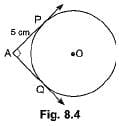RS Aggarwal Test: Circles - Question 2

### A circle touches x-axis at A and y-axis at B. If O is origin and OA = 5 units, then diameter of the circle is

Detailed Solution for RS Aggarwal Test: Circles - Question 2

OA = OB ⇒ OB = 5
⇒ OACB is a square.
⇒ AC = OA = 5
⇒ Diameter =10 units.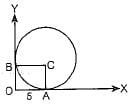RS Aggarwal Test: Circles - Question 3

### At point A on a diameter AB of a circle of radius 10 cm, tangent XAY is drawn to the circle. The length of the chord CD parallel to XY at a distance 16 cm from A is

Detailed Solution for RS Aggarwal Test: Circles - Question 3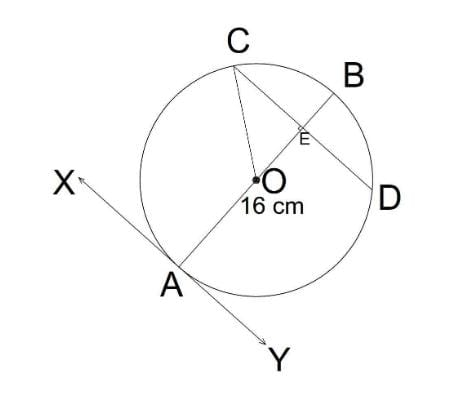In the given figure

XY is the tangent to the circle

We know that tangent is at right angle with the line joining the tangent point to the centre of the circle

Therefore,

∠OAY = 90°

∵ CD is parallel to XY

Therefore,

∠OAY = ∠BED = 90° (Corresponding angles)

Again,

Given that

AE = 16 cm

And the radius of the circle is 10 cm

Therefore,

AO = 10 cm

∴ OE = AE - AO = 16 - 10 = 6 cm

Also,

OC = 10 cm (Radius of the circle)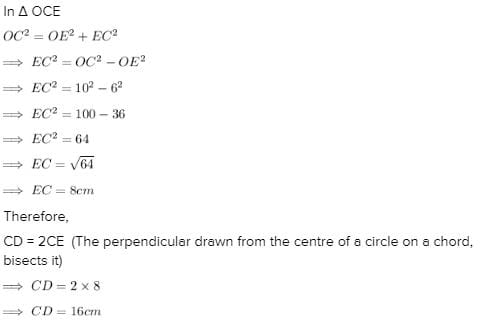RS Aggarwal Test: Circles - Question 4

From a point P which is at a distance of 13 cm from the centre O of a circle of radius 5 cm, the pair of tangents PQ and PR to the circle are drawn. Then the area of the quadrilateral PQOR is

Detailed Solution for RS Aggarwal Test: Circles - Question 4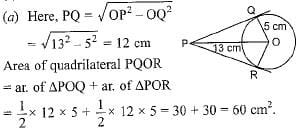RS Aggarwal Test: Circles - Question 5

Two circles touch each other externally at C and AB is common tangent of circles, then ∠ACB is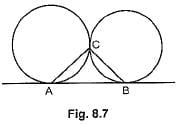RS Aggarwal Test: Circles - Question 6

In figure AT is a tangent to the circle with centre O such that OT = 4 cm and ∠OTA = 30°. Then AT is equal to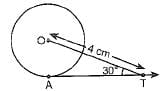Detailed Solution for RS Aggarwal Test: Circles - Question 6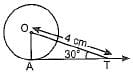∠OAT = 90° [∵ Tangent and radius are ⊥ to each other at the point of contact
In right-angle ΔOAT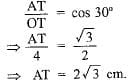RS Aggarwal Test: Circles - Question 7

In Fig. 8.8, if PA anti PB are tangents to the circle with centre O such that ∠APB = 50°, then ∠OAB is equal to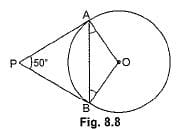RS Aggarwal Test: Circles - Question 8

In figure if O is centre of a circle, PQ is a chord and the tangent PR at P makes an angle of 50° with PQ, then ∠POQ is equal to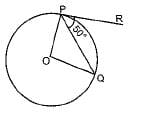Detailed Solution for RS Aggarwal Test: Circles - Question 8

OP ⊥ PR [∵ Tangent and radius are ⊥ to each other at the point of contact]
∠OPQ = 90° - 50° = 40°
∴ ∠OPQ = ∠OQP = 40°
In ΔOPQ,
⇒ ∠POQ + ∠OPQ + ∠OQP = 180°
⇒ ∠POQ + 40° + 40° = 180°
∠POQ = 180° - 80° = 100°.

RS Aggarwal Test: Circles - Question 9

If ΔABC is circumscribing a circle in the Fig. 8.12. The length of AB is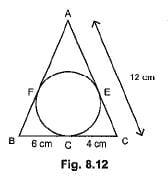RS Aggarwal Test: Circles - Question 10

From a point X, the length of the tangent to a circle is 20 cm and the distance of X from the centre is 25 cm. The radius of the circle is

## Mathematics (Maths) Class 10

115 videos|478 docs|129 tests
Information about RS Aggarwal Test: Circles Page
In this test you can find the Exam questions for RS Aggarwal Test: Circles solved & explained in the simplest way possible. Besides giving Questions and answers for RS Aggarwal Test: Circles, EduRev gives you an ample number of Online tests for practice

## Mathematics (Maths) Class 10

115 videos|478 docs|129 tests